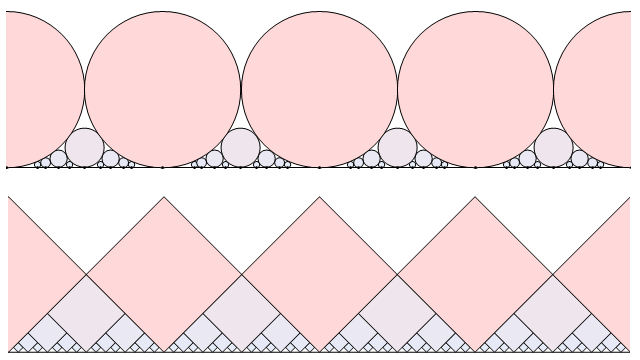# Closed-form bijections

One of the qualities of a quintessential British mathematician is the ability to invent crazy and contrived functions to satisfy particular properties. For instance, John Conway’s base-13 function was designed as a counter-example to the converse of the Intermediate Value Theorem. Another example is when most of the British RMM 2011 team found functions f and g over the reals such that f(g(x)) is strictly increasing and g(f(x)) is strictly decreasing.

Later, I received news that someone was trying to find a closed-form bijection between the integers and the rationals. By ‘closed-form’, you need to be able to express z (an arbitrary integer) in terms of q (an arbitrary rational) using only well-known standard functions, and vice-versa. You’re not allowed infinite series, infinite products or anything else that would require an infinite amount of paper when expanded. Similarly, it’s considered cheating to use an irrational number you’ve defined yourself — but pi, e, phi etc. are all permissible.

I challenge you to find a significantly simpler closed-form bijection than the one presented below:

Yes, it’s pretty hideous. It boils down to the idea that any positive dyadic rational can be uniquely expressed in the form n*2^k where n is an odd natural number and k is an integer. Similarly, any positive integer can be uniquely expressed in the form n*2^k where n is an odd natural number and k is a non-negative integer. Together with a closed-form bijection between integers and non-negative integers, we have a way to interchange between positive integers and positive dyadic rationals.

The kneading function is actually a pair of functions: one which maps integers to non-negative integers, and one which reverses the process. I have chosen the bijection between the ordered lists {0,1,2,3,4,5,6,…} and {0,-1,1,-2,2,-3,3,…}. The 2-adic valuation function, v2(x), gives the largest integer y such that 2^y divides x.

Using the sgn() and abs() functions, we can extend this to a closed-form bijection between integers and dyadic rationals. It is an odd function, meaning that if q and z are paired in the bijection, then so are -q and -z. Hence, 0 maps to itself. We are now extremely close to fulfilling the task of bijecting between the integers and rationals; we just require a function to map the rationals to the dyadic rationals.

Fortunately, one already exists. The Minkowski question mark function is an amazing beast, which maps rationals to dyadic rationals. More impressive is the fact that it maps quadratic surds (roots of quadratic equations with integer coefficients) to rationals.There are certain similarities between the rationals and dyadic rationals. The Ford circles (above) are defined by placing a circle of diameter 1/q² on each rational point p/q on the real line. Similarly, we can define the Ford diamonds (not an official term; I’m not sure if one exists) by placing a rotated square of diagonal length 1/2^k on each dyadic rational point p/2^k on the real line.

Applying the Minkowski question mark function to the Ford circles gives a deformed version of the Ford diamonds. Similarly, the inverse Minkowski question mark function should deform the Ford circles into a set of weird shapes positioned on the quadratic surds. Squircles, possibly? I have yet to experiment with this, but I’ll let you know if I find anything interesting.

This entry was posted in Uncategorized. Bookmark the permalink.

### 0 Responses to Closed-form bijections

1. Pingback: Digest | Complex Projective 4-Space

2.aaron meyerowitz says:

I stumbled on this old post. Here is a nice bijection which I am sure is not new. A positive integer is uniquely 2^a 3^b 5^c 7^d 11^e etc where the exponents are non-negative integers (and 0 from some point on.) A positive rational is uniquely 2^a 3^b 5^c 7^d 11^e etc where the exponents are integers (and 0 from some point on.) To get the rational corresponding to an integer change each prime power p^n to p^m where m=n/2 for n even and -(n+1)/2 for n odd. That is to say, the nth thing in the sequence -1,1,-2,2,-3,3,… That should work pretty well with 0 going to 0 and negatives to negatives.